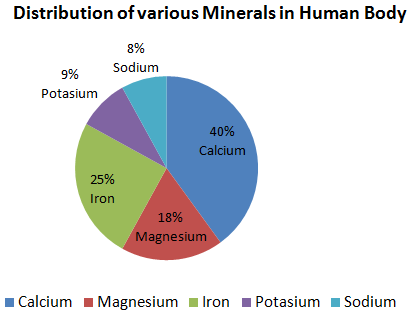Question 10

#The given graph shows the distribution of minerals in the human body.Which two minerals are required almost in the same percentage for the human body?

Solution

Given the distribution of minerals in the human body

Calcium=40%

Magnesium=18%

Iron=25%

sodium=9%

potassium=8%

from this show that percentage of sodium nearly equal to potassium